# OECD Trust in government data analysis 8 - Trust is government cannot be explained simply by per capita GDP, inflation and long-term unemployment.Photo by Rob Wicks on Unsplash

This post is following of above post. In the previous post I used only JPN data.
Let's use whole data in this post.
Firstly,  I make a multiple linear regression model by year. Dependent variable is trut: Trust in governmnet, independent variables are l_capi: log(per capita GDP), cpi: cpi growth rate, inflation rate and long_unemp: long term unemployment rate.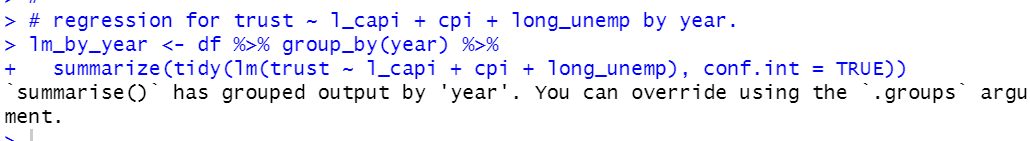Let's see l_capi coefficients.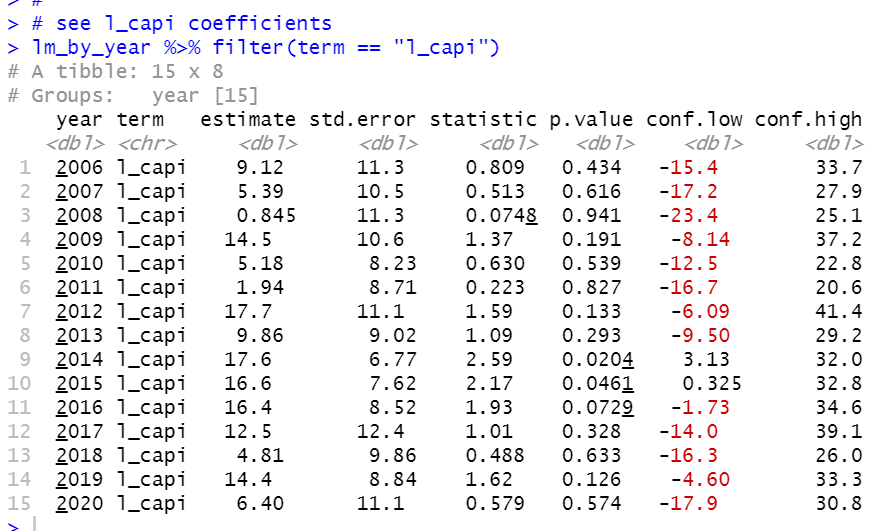All year, coefficients are positive value, but only 2014 and 2015 are statistically different from 0.

Let's see cpi.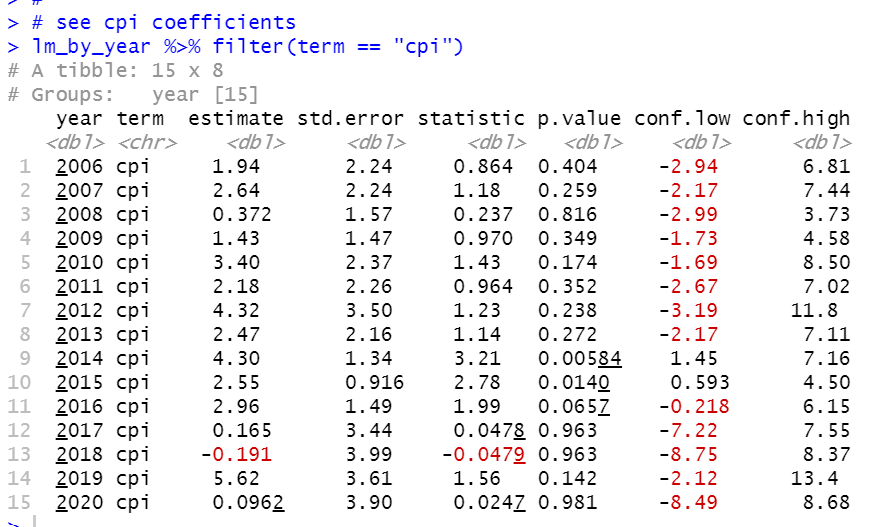2018 is negative coefficient but not statistically different from 0. Again, 2014 and 2015 has statistically different from 0.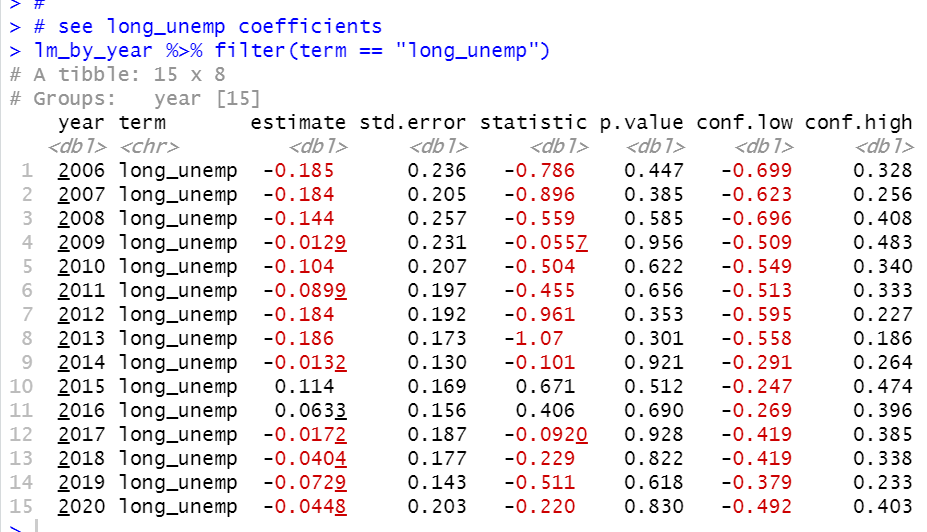There is no year which has statistically significant coeffcient.

Next, I make linear regression model by iso.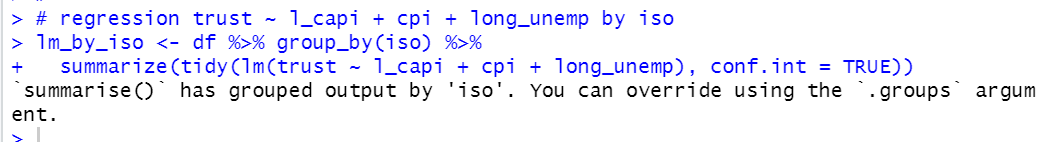Let's see l_capi coefficient.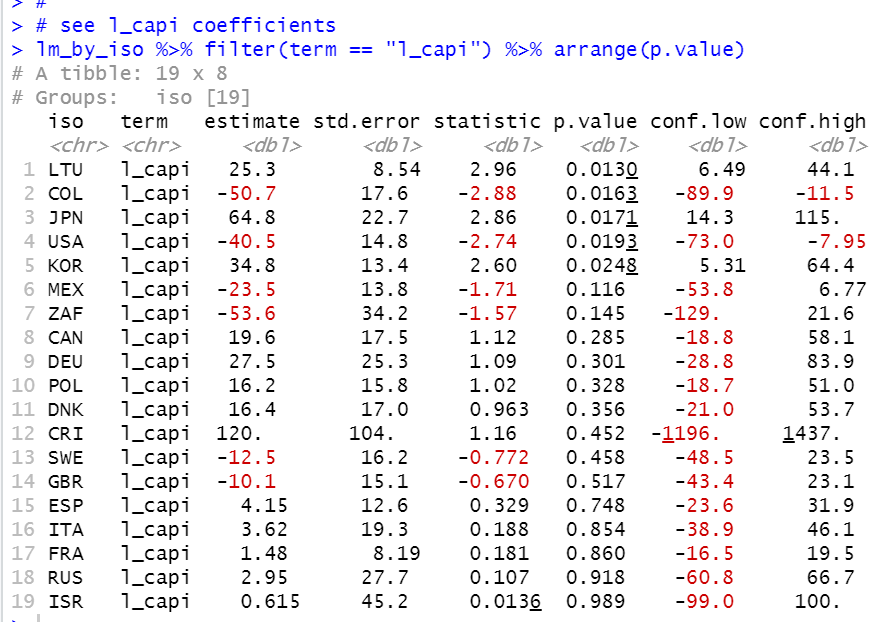LTU, COL, JPN, USA and KOR have statistically significant coefficient value, but COL and USA have nagative while LTU, JPN and KOR have positive value.

Let's see cpi.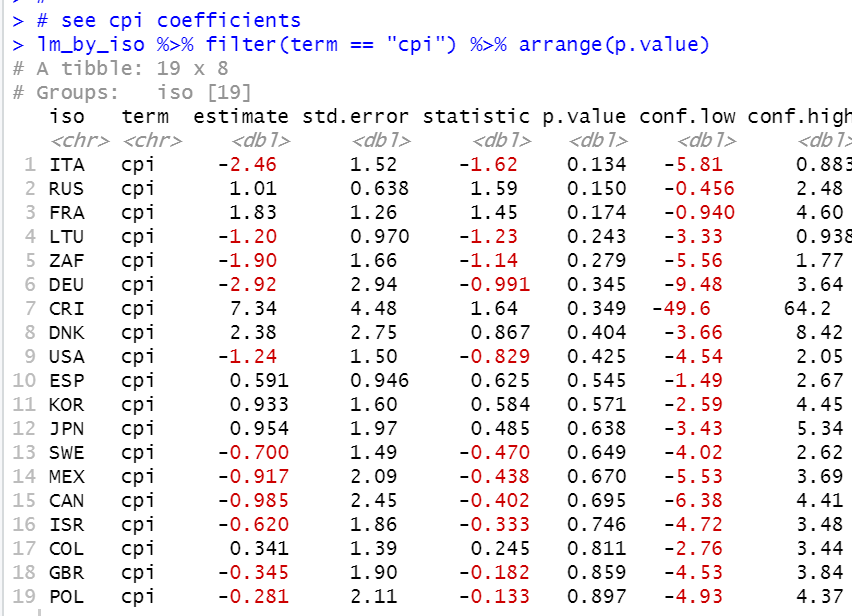All iso don't have statistically significant coefficients from 0.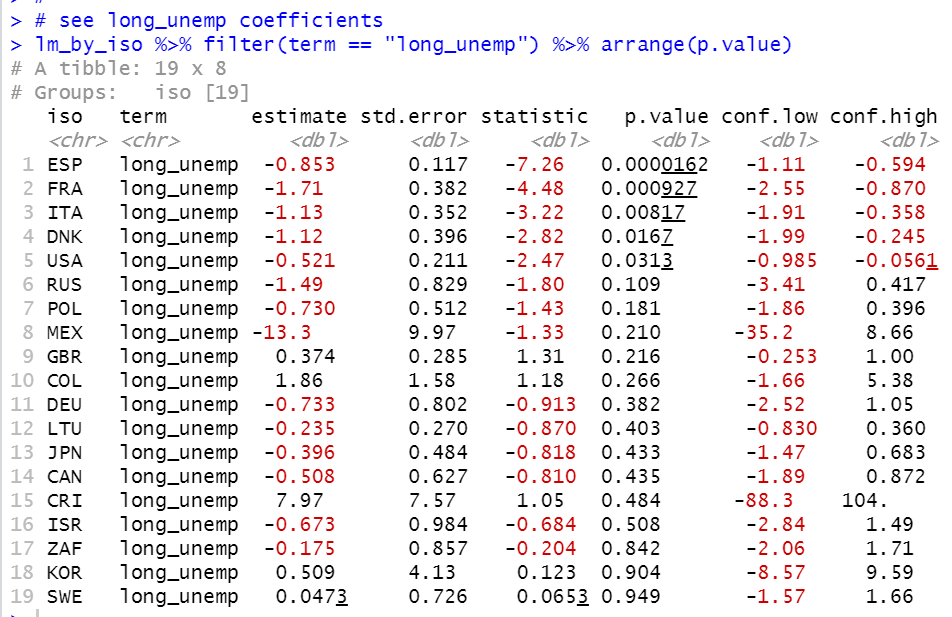ESP, FRA, ITA, DNK and USA have statistically significant coefficient and they are all negative value. It seems make sense, the lower longterm unemployment, the higher trust in governmnet.

Trust in government cannot be explained simply by per capita GDP, inflation rate and longterm unemployment.

That's it. Thank you!